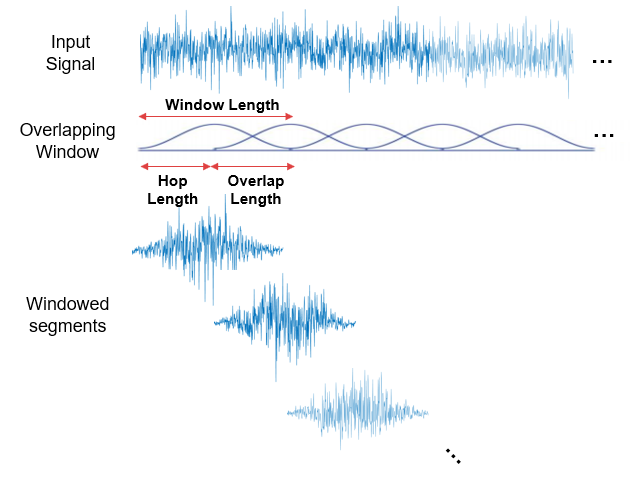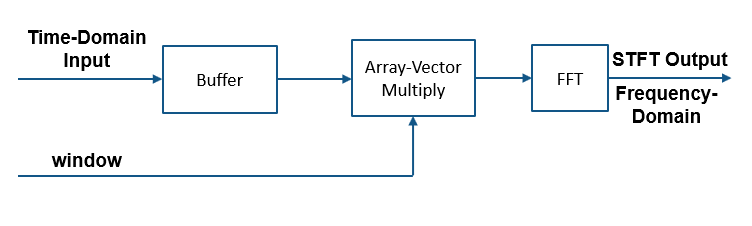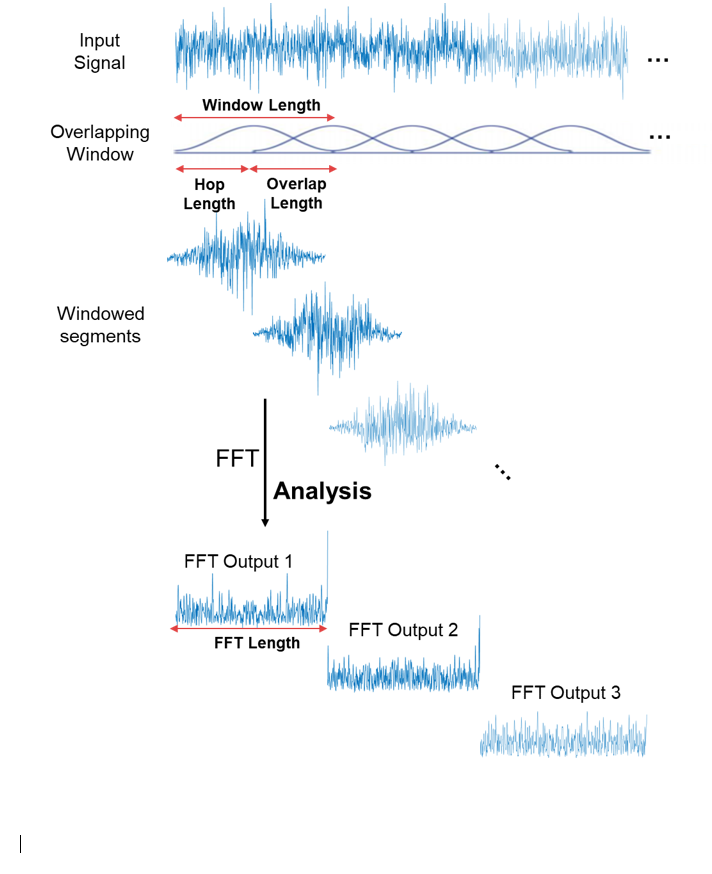dsp.STFT

Short-time FFT

Description

The dsp.STFT object computes the short-time Fourier transform (STFT) of the time-domain input signal. The object accepts frames of time-domain data, buffers them to the desired window length and overlap length, multiplies the samples by the window, and then performs FFT on the buffered windows. For more details, see Algorithms.

Use the STFT to analyze the frequency content of a signal that varies with time.

Creation

Description

stf = dsp.STFT returns an object, stf, that implements the short-time FFT. The object processes the data independently across each input channel over time.

stf = dsp.STFT(window) returns a short-time FFT object with the Window property set to window.

stf = dsp.STFT(window,overlap) returns a short-time FFT object with the Window property set to window and the OverlapLength property set to overlap.

example

stf = dsp.STFT(window,overlap,nfft) returns a short-time FFT object with the Window property set to window, the OverlapLength property set to overlap, and the FFTLength property set to nfft.

stf = dsp.STFT(Name,Value) returns a short-time FFT object with each specified property name set to the specified value. You can specify additional name-value pair arguments in any order.

Properties

expand all

Analysis window, specified as a vector of real elements.

The object buffers the input into overlapping window segments using the specified window length and overlap length, and then multiplies each overlapped segment by the window.Tunable: Yes

Data Types: single | double

Number of samples by which consecutive windows overlap, specified as a positive integer. The window overlap reduces the artifacts at the data boundaries.Data Types: single | double | int8 | int16 | int32 | int64 | uint8 | uint16 | uint32 | uint64

FFT length, specified as a positive integer. This property determines the length of the STFT output (number of rows). The FFT length must be greater than or equal to the window length.

Data Types: single | double | int8 | int16 | int32 | int64 | uint8 | uint16 | uint32 | uint64

Frequency range over which the short-time FFT is computed, specified as:

• 'twosided' ––The short-time FFT is computed for complex or real inputs signals. The length of the short-time FFT is equal to the value you specify in the FFTLength property.

• 'onesided' –– The one-sided short-time FFT is computed for real input signals only. When the FFT length is even, the short-time FFT length is FFTLength/2+1. If FFT length is odd, the length of the short-time FFT is equal to (FFTLength+1)/2.

Usage

Description

example

y = stf(x) applies short-time FFT on the input x and returns the frequency-domain output y.

Input Arguments

expand all

Time-domain input signal, specified as a vector or a matrix. If the input is a matrix, the object treats each column as an independent channel. The frame size (number of rows in x) must be equal to or less than the hop length (window length − overlap length).

The input can be a variable-sized signal. That is, the frame size of the signal can change in between calls to the object algorithm without calling the release function. The number of channels must remain the same.

If the FrequencyRange property is set to 'onesided', the input must be real. If the FrequencyRange property is set to 'twosided', the input can be real or complex.

Data Types: single | double
Complex Number Support: Yes

Output Arguments

expand all

Short-time FFT output, returned as a vector or a matrix.

If there are enough samples (equal to hop length) to form an STFT output, y is an FFTLength-by-N matrix, where N is the number of input channels. If there are not enough samples to form an STFT output, y is empty.

The data type of the output matches that of the input signal.

Data Types: single | double
Complex Number Support: Yes

Object Functions

 step Run System object algorithm release Release resources and allow changes to System object property values and input characteristics reset Reset internal states of System object clone Create duplicate System object isLocked Determine if System object is in use getFrequencyVector Get the vector of frequencies at which the short-time FFT is computed

Examples

collapse all

Short-time spectral attenuation is achieved by applying a time-varying attenuation to the short-time spectrum of a noisy signal. The gain of the attenuation is determined by the estimate of the noise power in each subband of the spectrum. This gain, when applied to the noisy spectrum, attenuates the subbands with higher noise power and lifters the subbands with lesser noise power.

Here are the steps involved in performing the short-time spectral attenuation:

1. Analyze the noisy input signal by computing the short-time Fourier transform (STFT).

2. Multiply each subband of the transformed signal with a real positive gain less than 1.

3. Synthesize the denoised subbands by taking the inverse short-time Fourier transform (ISTFT). The resconstructed signal is the denoised input signal.

Use the dsp.STFT and dsp.ISTFT objects to compute the short-time and the inverse short-time Fourier transforms, respectively.

Noisy Input Signal

The input is an audio signal sampled at the 22,050 Hz. The dsp.AudioFileReader object reads this signal in frames of 512 samples. The audio signal is corrupted by white Gaussian noise that has a standard deviation of 0.05. Use the audioDeviceWriter object to play the noisy audio signal to your computer's audio device.

FrameLength = 512;
'SamplesPerFrame',FrameLength);

noiseStd = 0.05;
while ~isDone(afr)
cleanAudio = afr();
noisyAudio = cleanAudio + noiseStd * randn(FrameLength,1);
end
reset(afr)

Initialize Short-Time and Inverse Short-Time Fourier Transform Objects

Initialize the dsp.STFT and dsp.ISTFT objects. Set the window length equal to the input frame length and the hop length to 16. The overlap length is the difference between the window length and the hop length, OL = WLHL. Set the FFT length to 1024.

WindowLength = FrameLength;
HopLength = 16;
numHopsPerFrame = FrameLength / 16;
FFTLength = 1024;

The window used to compute the STFT and ISTFT is a periodic hamming window with length 512. The ConjugateSymmetricInput flag of the istf object is set to true, indicating that the output of the istf object is a conjugate-symmetric signal.

win = hamming(WindowLength,'periodic');
stf = dsp.STFT(win,WindowLength-HopLength,FFTLength);
istf = dsp.ISTFT(win,WindowLength-HopLength,1,0);

Gain Estimator

The next step is to define the gain estimator parameters. This gain is applied to the noisy spectrum to attenuate the subbands with higher noise power and lifter the subbands with lesser noise power.

dec = 16;
alpha = 15;
stftNorm = (sum(win.*win) / dec).^2;

Spectral Attenuation

Feed the audio signal to stf one hop-length at a time. Apply the estimated gain to the transformed signal. Reconstruct the denoised version of the original speech signal by performing an inverse Fourier transform on the individual frequency bands. Play the denoised audio signal to the computer's audio device.

while ~isDone(afr)
cleanAudio =  afr();
noisyAudio = cleanAudio + noiseStd * randn(FrameLength,1);
y = zeros(FrameLength,1); % y holds the denoised audio frame

% Feed audio to stft one hop-length at a time
for index = 1:numHopsPerFrame
X = stf(noisyAudio((index-1)*HopLength+1:index*HopLength));
% Gain estimator
Z = abs(X).^2 / (noiseStd^2 * alpha) / stftNorm;
Z(Z<=1) = 1;
Z = 1 - 1./Z;
Z = sign(Z) .* sqrt(abs(Z));
X = X .* Z;
% Convert back to time-domain
y((index-1)*HopLength+1:index*HopLength) = istf(X);
end
% Listen to denoised audio:
end

Perfect reconstruction is when the output of dsp.ISTFT matches the input to dsp.STFT. Perfect reconstruction is obtained if the analysis window, $\mathit{g}\left(\mathit{n}\right)$, obeys the constant overlap-add (COLA) property at hop-size R.

$\sum _{\mathit{m}=-\infty }^{\infty }\mathit{g}\left(\mathit{n}-\mathit{mR}\right)=1,\text{\hspace{0.17em}}\forall \mathit{n}\in \text{\hspace{0.17em}}Ζ\text{\hspace{0.17em}\hspace{0.17em}\hspace{0.17em}\hspace{0.17em}\hspace{0.17em}}\left(\mathit{g}\in \mathrm{COLA}\left(\mathit{R}\right)\right)$

A signal is perfectly reconstructed if the output of the dsp.ISTFT object matches the input to the dsp.STFT object.

iscola Function

The iscola function checks that the specified window and overlap satisfy the COLA constraint to ensure that the inverse short-time Fourier transform (ISTFT) results in perfect reconstruction for non-modified spectra. The function returns a logical true if the combination of input parameters is COLA-compliant and a logical false if not. The method argument of the function is set to 'ola' or 'wola' depending on whether the inversion method uses weighted overlap-add (WOLA).

Check if hann() window of length 120 samples and an overlap length of 60 samples is COLA compliant.

winLen = 120;
overlapLen = 60;
win = hann(winLen,'periodic');
tf = iscola(win,overlapLen,'ola')
tf = logical
1

Initialization

Initialize the dsp.STFT and dsp.ISTFT System objects with this hann window that is COLA compliant. Set the FFT length to equal the window length.

frameLen = winLen-overlapLen;
stf = dsp.STFT('Window',win,'OverlapLength',overlapLen,'FFTLength',winLen);

Reconstruct Data

Compute the STFT of a random signal. Set the length of the input signal to equal the hop length (window length – overlap length). Since the window is COLA compliant, the ISTFT of this non-modified spectra perfectly reconstructs the original time-domain signal.

To confirm, compare the input, x to the reconstructed output, y. Due to the latency introduced by the objects, the reconstructed output is shifted in time compared to the input. Therefore, to compare, take the norm of the difference between the reconstructed output, y and the previous input, xprev. The norm is very small, indicating that the output signal is a perfectly reconstructed version of the input signal.

n = zeros(1,100);
xprev = 0;
for i = 1:100
x = randn(frameLen,1);
X = stf(x);
y = istf(X);
n(1,i) = norm(y-xprev);
xprev = x;
end
max(abs(n))
ans = 1.6972e-13

In WOLA, a second window called the synthesis window, $\mathit{f}\left(\mathit{n}\right)$, is applied after the IFFT operation and before overlap-add. The synthesis and analysis windows are typically identical and are usually obtained by taking the square root of windows satisfying COLA (thereby ensuring perfect reconstruction).

iscola Function

Check if sqrt(hann()) window of length 120 samples and an overlap length of 60 samples is WOLA compliant. Set the method argument of the iscola function to 'wola'. The output of the iscola function is 1 indicating that this window is WOLA compliant.

winWOLA = sqrt(hann(winLen,'periodic'));
tfWOLA = iscola(winWOLA,overlapLen,'wola')
tfWOLA = logical
1

Reconstruct Data with WOLA

Release the dsp.STFT and dsp.ISTFT System objects and set the window to sqrt(hann(winLen,'periodic')) window. To use weighted overlap-add on the ISTFT side, set the 'WeightedOverlapAdd' to true.

release(stf);
release(istf);
stf.Window = winWOLA;
istf.Window = winWOLA;

n = zeros(1,100);
xprev = 0;
for i = 1:100
x = randn(frameLen,1);
X = stf(x);
y = istf(X);
n(1,i) = norm(y-xprev);
xprev = x;
end
max(abs(n))
ans = 4.6664e-15

The norm of the difference between the input signal and the reconstructed signal is very small indicating that the signal has been reconstructed perfectly.

expand all

Algorithms

Here is a sketch of how the algorithm is implemented:The time-domain input signal is buffered based on a user-specified window length (WL) and overlap length (OL). The hop size, R, is defined as R = WLOL. Buffered windows are multiplied by a user-specified window of length WL. The STFT output is the FFT of this product. The number of time-domain samples required to form a new FFT output is R.

Here is an illustration of how a random signal looks like in the original time-domain, after multiplying with the overlapping windows, and after applying FFT on the multiplied windows:Allen, J.B., and L. R. Rabiner. "A Unified Approach to Short-Time Fourier Analysis and Synthesis,'' Proceedings of the IEEE, Vol. 65, pp. 1558–1564, Nov. 1977.

DSP System Toolbox DocumentationGet trial now